### Vol 17, No 5 (2017) / Wang

Classification of stellar populations in globular clusters

# Classification of stellar populations in globular clusters

Wang Yue1, 2, Zhao Gang1, 2, , Li Hai-Ning1

Key Laboratory of Optical Astronomy, National Astronomical Observatories, Chinese Academy of Sciences, Beijing 100012, China
School of Astronomy and Space Science, University of Chinese Academy of Sciences, Beijing 100049, China

† Corresponding author. E-mail: gzhao@nao.cas.cn

Abstract: Abstract

Possessing multiple stellar populations has been accepted as a common feature of globular clusters (GCs). Different stellar populations manifest themselves with different chemical features, e.g. the well-known O−Na anti-correlation. Generally, the first (primordial) population has O and Na abundances consistent with those of field stars with similar metallicity; while the second (polluted) population is identified by their Na overabundance and O deficiency. The fraction of the populations is an important constraint on the GC formation scenario. Several methods have been proposed for the classification of GC populations. Here we examine a criterion derived based on the distribution of Galactic field stars, which relies on Na abundance as a function of [Fe/H], to distinguish first and second stellar populations in GCs. By comparing the first population fractions of 17 GCs estimated by the field star criterion with those in the literature derived by methods related to individual GCs, we find that the field star criterion tends to overestimate the first population fractions. The population separation methods, which are related to an individual GC sample, are recommended because the diversity of GCs can be taken into consideration. Currently, more caution should be exercised if one wants to regard field stars as a reference for the identification of a GC population. However, further study on the connection between field stars and GCs populations is still needed.

Keywords: stars: abundances;globular clusters: general

## 1 Introduction

Globular clusters (GCs) have been regarded for a long time as being composed of a single stellar population whose stars have consistent age and chemical composition. In recent years, the large amount of observations, both photometric and spectroscopic, have revealed that GCs have multiple (at least two) stellar populations and it should be a common feature of GCs (e.g. Carretta et al. ; Milone et al. ). While the (primordial) first population (1P) of stars has abundances consistent with field stars with similar metallicity, the (polluted) second population (2P) of stars is recognized by their Na overabundance and O depletion, based on the well-known O−Na anti-correlation.

However, the formation of multiple populations is still being debated. Although several scenarios have been proposed, e.g. the most commonly-invoked massive asymptotic giant branch (AGB) star scenario (e.g. D’Ercole et al. ; Ventura et al. ) and fast-rotating massive star (FRMS) scenario (e.g. Krause et al. ), none of them can explain all the observed phenomena associated with GCs (Renzini et al. ).

The fractions of different stellar populations in GCs are important constraints on the GC formation scenario, since they are closely related to the initial masses of GCs. As estimated by Carretta et al. (), the 1P stars account for 33%, on average, in the GCs they studied. This fraction implies that more than 95% of the 1P stars were lost from GCs and the initial stellar masses could be 8−25 times larger than today (Decressin et al. , ; Carretta et al. ; D’Ercole et al. ; Schaerer & Charbonnel ).

So far, several methods to distinguish different GC populations have been proposed, including both chemical and photometric ones. Carretta et al. () defined a critical Na abundance of [Na/Fe]cri = [Na/Fe]min + 0.3 dex, where [Na/Fe]min is the minimum value of [Na/Fe] derived for the entire sample of a given GC. García-Hernández et al. () used an extreme-deconvolution (XD) method, fitting the distribution of elemental abundances as a sum of two Gaussian populations representing 1P and 2P, to identify 1P and 2P stars. MacLean et al. () adopted their population separation point in such a way that the [Na/O] value of that point corresponds to the least number of stars in between two stellar populations. Moreover, taking advantage of high-precision HST photometry, Milone et al. () identified multiple stellar populations for GCs with the aid of a pseudo two-color diagram (or ‘chromosome map’) where GC stars are distributed along two main, distinct groups that correspond to the two populations. All these methods above refer to the stellar samples of individual GCs. However, Carretta () studied the population fractions for NGC 6752 by comparing cluster stars with field stars that have similar metallicity in the [Fe/H]−[Na/H] plane, which is a method following the definition of the two stellar populations.

In order to investigate how the two sets of GC population classification methods compare, i.e. by referring to individual GC sample and to field stars, we extend the method of Carretta () to the whole metallicity range of Galactic GCs, and compare the derived GC population fractions to those estimated by the methods where individual GC samples are considered.

This paper is organized as follows: in Section 2 we describe the derivation of the criterion for GC population classification from Galactic field stars; then the derived criterion is applied to GCs in Section 3, where a comparison with other methods is also presented; finally the summary and conclusion close the paper in Section 4.

## 2 Field star criterion for GC population classification

Carretta () assembled a dataset of 1891 Milky Way field stars (a majority of which was dwarfs but also including giants) from the literature in the metallicity range of [Fe/H]=−3.15∼ 0.48 dex, with Na abundance derived from high resolution spectra. The whole sample was homogenized in [Fe/H] and [Na/H] to a scale defined by the reference sample of Gratton et al. (). Here, we take advantage of this field star sample and aim to find a 1P−2P separation criterion based on the Na abundance distribution of field stars covering the whole metallicity range of Galactic GCs.

Figure 1 shows the [Fe/H]−[Na/H] distribution of field stars. To analyze the trend quantitatively, we first divided the stars into 14 bins as a function of [Fe/H] with a bin width of 0.2 dex. The median [Fe/H] and [Na/H] of each bin were calculated and are shown as filled yellow circles in the figure with error bars representing the scatter (standard deviation) of the [Na/H] in each bin. By viewing the [Fe/H]−[Na/H] distribution of the whole sample, we roughly distinguished four regions of [Fe/H] (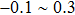,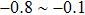,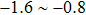and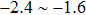dex) in which the [Fe/H]−[Na/H] distributions had different trends (i.e. slopes and scatters). We applied a linear fit to the median [Fe/H]−[Na/H] data points in each region, taking the scatters into consideration. The derived fits are shown by dashed lines with different colors for different regions, and the coefficients of the linear fits are listed in Table 1.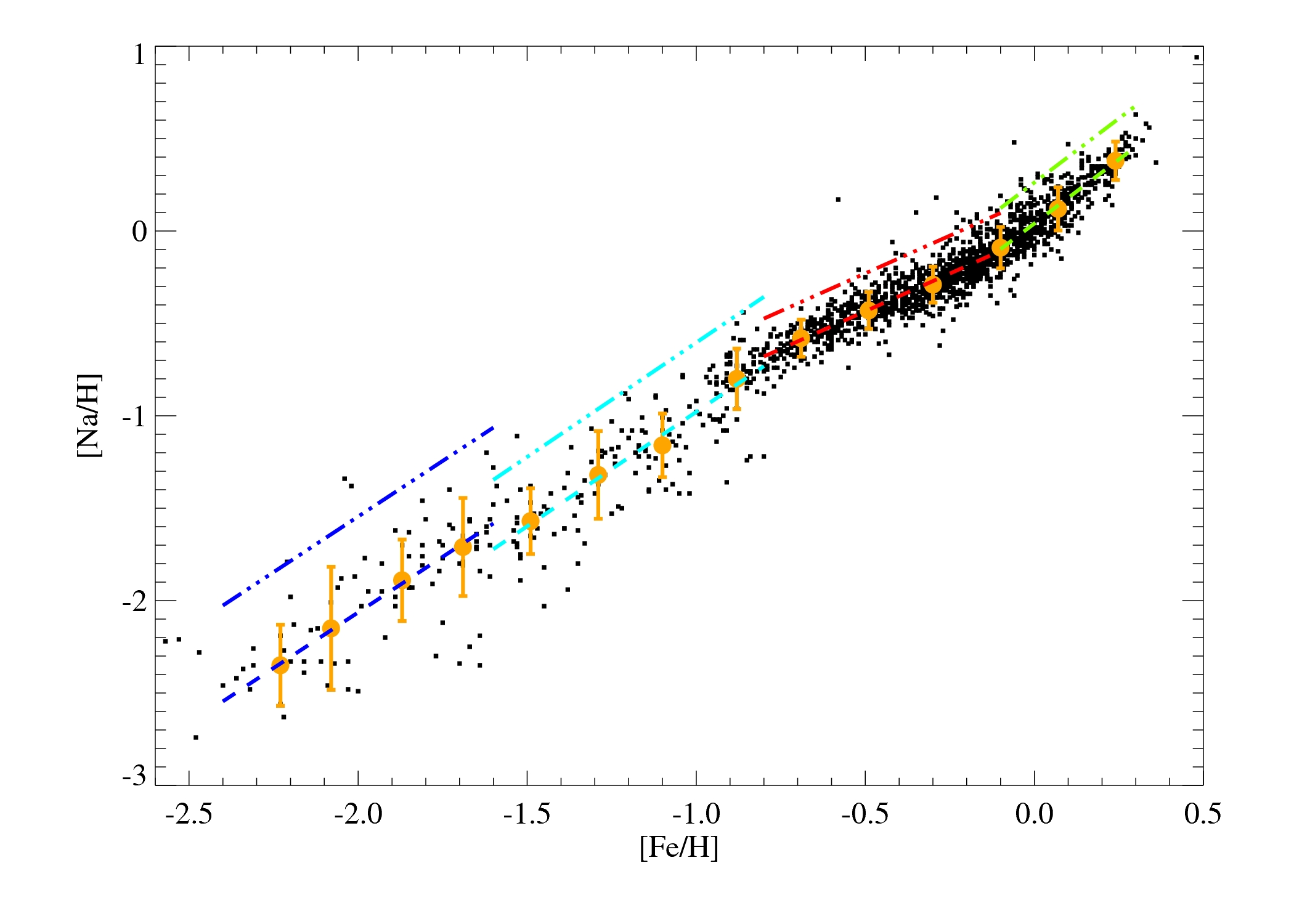Fig. 1 The [Fe/H]-[Na/H] distribution of field stars. Black dots represent field stars. Filled yellow circles represent the median [Fe/H] and [Na/H] of each bin, with the associated error bars being the scatter in [Na/H]. Dashed lines correspond to linear fits of the distributions in four regions, and dash-dotted lines correspond to the linear fits plus 2σ which represent the upper bound of the distribution.
Region of [Fe/H] a b c
(dex)
−0.1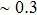1.386 0.041 0.262
−0.8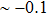0.816 –0.026 0.179
−1.6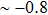1.238 0.259 0.634
−2.4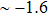1.203 0.343 0.862

Table 1 Coefficients of the linear fits for the [Fe/H]−[Na/H] distribution of the field star sample, and those of the criterion for GC population classification. The linear fit is in the format of [Na/H] = a*[Fe/H] + b, while the criterion is expressed as [Na/H]cri = a*[Fe/H] + c.

We increased the linear fits of the distribution by 2σ (here σ was the mean value of the [Na/H] scatters of the bins in each region) to represent the upper bound on the [Na/H] distribution for the field stars. The derived upper bounds on the [Fe/H]−[Na/H] relations are shown by dash-dotted lines in Figure 1, and the coefficients of the function are listed in Table 1. Carretta () checked the field star sample used here carefully by eye, and found a critical [Na/H] value of −1.30 dex at the metallicity of NGC 6752 (−1.56 dex) for the population separation. His critical [Na/H] is exactly the same as the value derived from the above relation at the same [Fe/H], supporting the notion that our derived relation can well represent the upper bound of the [Na/H] distribution for field stars. According to the definition of the two GC populations that 1P stars have similar Na abundances compared to field stars while 2P stars show Na overabundance, we adopted these upper bound [Fe/H]−[Na/H] relations as the criterion to distinguish 1P and 2P GC stars: at a given [Fe/H], GC stars with Na abundance lower than the corresponding [Na/H] value of the relation ([Na/H]cri) are identified as 1P stars, while those with higher Na abundances belong to 2P.

## 3 Population fractions of globular clusters

To apply the 1P−2P separation criterion derived from field stars (‘field star criterion’), we took data on 17 GCs (NGC 104, NGC 288, NGC 1904, NGC 2808, NGC 3201, NGC 4590, NGC 5904, NGC 6121, NGC 6171, NGC 6254, NGC 6388, NGC 6397, NGC 6752, NGC 6809, NGC 6838, NGC 7078, NGC 7099), derived by Carretta et al. (, , ) from the homogeneous analysis of high-resolution FLAMES/GIRAFFE spectra of RGB stars, which are also on the same scale as the field star sample.

In Figure 2 we show the 17 GCs together with the field stars in the same [Fe/H]−[Na/H] plane. As expected, the bottom parts of the GC samples roughly overlap with the field stars, while those stars with higher [Na/H] should be regarded as 2P stars. Applying the field star criterion (dash-dotted lines) to the GC samples, we compute the 1P−2P separation Na abundance [Na/H]cri and derive the numbers and fractions of the two populations of stars for each GC. The [Na/H]cri values and the 1P star numbers and fractions are listed in Table 2. The errors associated with the fraction were computed from Poisson’s statistics. On average, a 1P fraction of ∼ 50% was derived.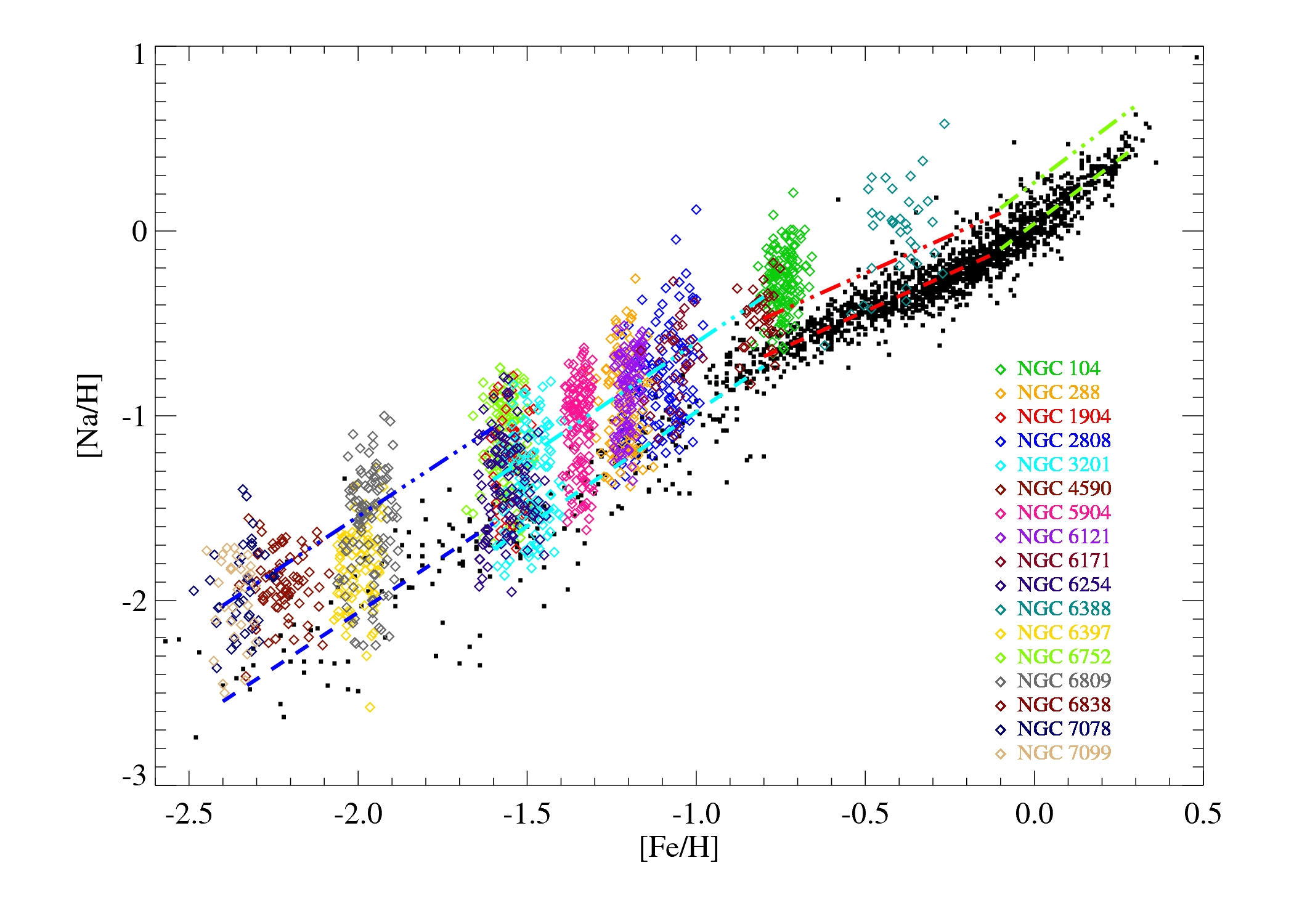Fig. 2 Na abundance distributions of 17 GCs compared to the field stars. The lines are the same as in Fig. 1.
NGC [Fe/H] [Na/H]cri Ntotal N1P F1P F1P,Carretta F1P,Milone
(1) (2) (3) (4) (5) (6) (7) (8)
104 −0.74 −0.42 147 22 0.15±0.03 0.27±0.05 0.175±0.009
288 −1.22 −0.88 96 53 0.55±0.08 0.33±0.07 0.542±0.031
1904 −1.54 −1.27 48 19 0.40±0.09 0.40±0.09
2808 −1.10 −0.73 121 77 0.64±0.07 0.50±0.07 0.232±0.014
3201 −1.50 −1.22 115 77 0.67±0.08 0.35±0.06 0.436±0.036
4590 −2.23 −1.82 73 53 0.73±0.10 0.40±0.09 0.381±0.024
5904 −1.35 −1.04 130 55 0.42±0.06 0.27±0.05 0.235±0.013
6121 −1.20 −0.85 103 43 0.42±0.06 0.30±0.06 0.285±0.037
6171 −1.07 −0.69 33 16 0.48±0.12 0.33±0.11 0.397±0.031
6254 −1.56 −1.30 109 67 0.61±0.08 0.38±0.07 0.364±0.028
6388 −0.41 −0.16 35 10 0.29±0.09 0.41±0.11 0.245±0.010
6397 −1.99 −1.53 85 77 0.91±0.10 0.25±0.13 0.345±0.036
6752 −1.56 −1.30 113 27 0.24±0.05 0.27±0.05 0.294±0.023
6809 −1.97 −1.51 115 66 0.57±0.07 0.20±0.05 0.311±0.029
6838 −0.81 −0.48 39 17 0.44±0.11 0.29±0.08 0.622±0.038
7078 −2.34 −1.95 40 19 0.47±0.11 0.39±0.11 0.399±0.019
7099 −2.36 −1.98 31 14 0.45±0.12 0.41±0.12 0.380±0.028

Table 2The 1P star numbers and fractions of the 17 GCs, together with the GC [Fe/H] and critical Na abundance [Na/H]cri. The 1P fractions derived by Carretta et al. () and Milone et al. () are listed in the 7th and 8th columns, respectively..

The 1P fractions estimated by Carretta et al. () are also listed in Table 2 in the 7th column, who applied the criterion of [Na/Fe]cri = [Na/Fe]min+0.3 dex, where [Na/Fe]min is the minimum value of [Na/Fe] derived for the entire sample of a given GC and 0.3 dex corresponds to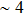times their error in [Na/Fe]. The top panel of Figure 3 shows the difference between the 1P fractions derived by the field star criterion and those by Carretta et al. () for the 17 GCs. The results by the field star criterion are on average 0.16 (dashed line) higher than those derived by Carretta et al. (), with a standard deviation (σ) of 0.19 (dash-dotted lines).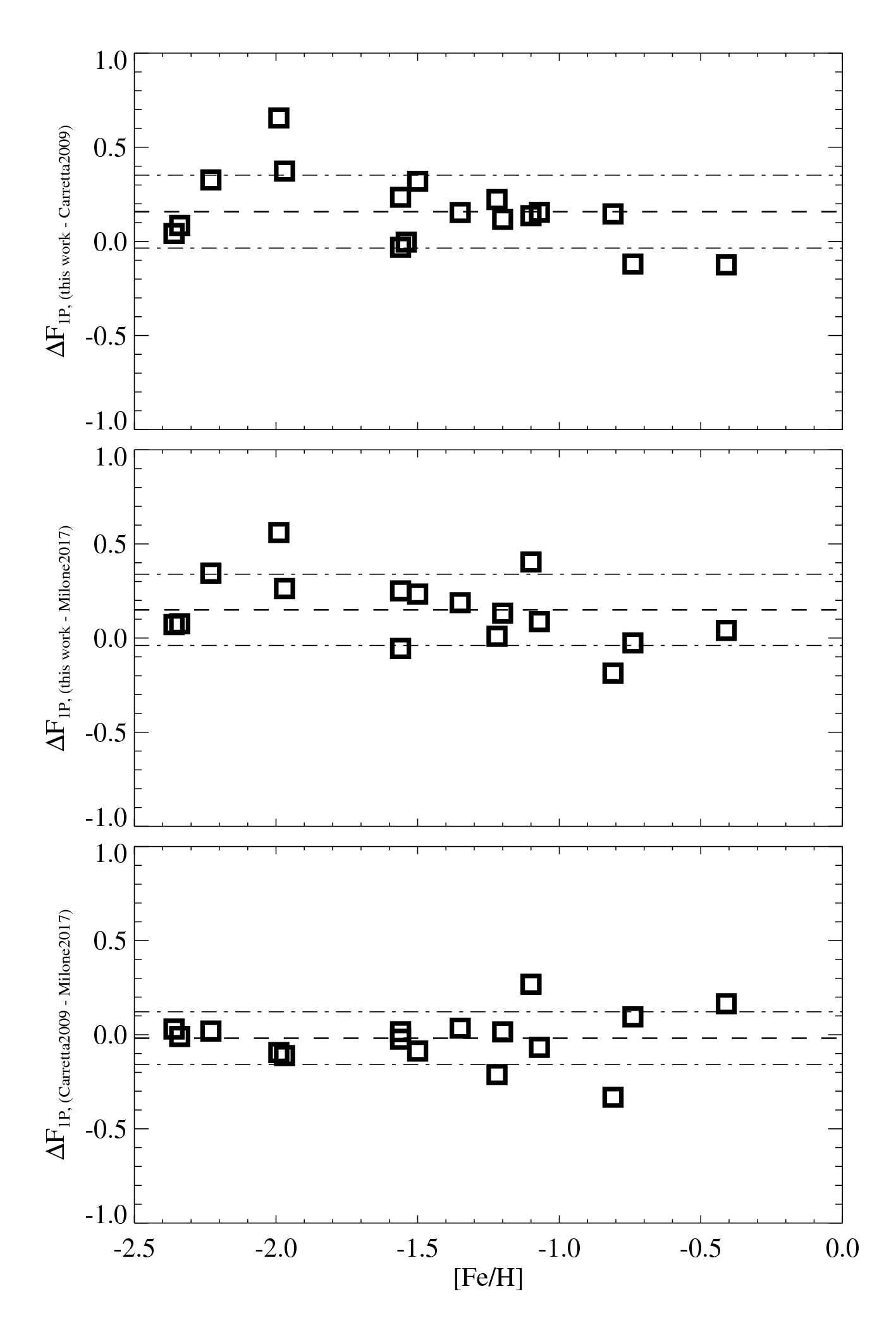Fig. 3 The difference of the estimated 1P fraction for the 17 GCs in this work (by the field star criterion), Carretta et al. () and Milone et al. (). The dashed line corresponds to the mean value of the difference, while the dash-dotted lines represent the mean ±1σ.

Milone et al. () used high-precision HST photometry of RGB stars in Galactic GCs to identify and characterize their multiple stellar populations. Taking advantage of their pseudo two-color diagram (or ‘chromosome map’) where cluster stars are distributed along two major, distinct groups that correspond to the two populations, they estimated the 1P star fractions for their sample of GCs.

In Table 2 we list their results in the 8th column. A comparison of the 1P fractions derived by the field star criterion and by Milone et al. () is shown in the middle panel of Figure 3. The 1P fractions estimated based on the field star criterion are on average 0.15 (dashed line) higher than those derived by Milone et al. (), with a standard deviation (σ) of 0.19 (dash-dotted lines). This difference is quite consistent with the difference between the fractions by the field star criterion and those by Carretta et al. ().

If we compare the 1P fractions derived chemically by Carretta et al. () and photometrically by Milone et al. (), as shown in the bottom panel of Figure 3, consistent results between the two studies are found, with a mean difference of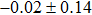where the value of Milone et al. () is negligibly higher. Their agreement could result from the fact that the two works both refer to an individual GC sample when classifying 1P−2P stars, but not rely on any other (uniform) reference. Although chemical and photometric methods are used respectively, the connection between stellar chemical and photometric features has been confirmed by several studies, e.g. as shown by Milone et al. (, figure 9), the photometrically identified 1P stars indeed have primordial chemical composition, while 2P stars are Na-rich and O-poor.

From comparison of the three pairs of 1P fraction data sets in Figure 3, we identified four outlier GCs, i.e. in two out of the three comparisons they show a difference which is larger than 1σ from the corresponding mean difference (namely located out of the dash-dotted lines). These include NGC 2808, NGC 6388, NGC 6397 and NGC 6838. For NGC 6838, the sample from Carretta et al. () is not evenly distributed in [Na/H] and few stars are presented at the low-Na end. It might be a consequence of the small sample size and/or uneven sampling, and leads to a low 1P fraction derived by Carretta et al., and also by the field star criterion, compared to the value derived photometrically by Milone et al. ().

NGC 6388 and NGC 2808 both have very complex stellar populations as indicated by their behaviors in terms of photometric data. In the study of Milone et al. (), NGC 6388 is found to be a Type II GC whose chromosome map exhibits extra 1P and 2P sequences. Milone et al. () has found that NGC 2808 contains at least five stellar populations, which have different contents of light elements and helium. The complex populations of NGC 2808 can also be recognized by its chromosome map (Milone et al. ). The cases of these two peculiar GCs indicate that the complexity of a GC in terms of multiple stellar populations could make it more difficult to use different methods to derive similar/consistent population fractions.

When taking the field stars as a reference to classify GC populations, the derived 1P fraction is systematically higher compared to those estimated through the methods where only the GC sample itself is concerned. NGC 6397 shows the most deviation among the comparison sample, for which the field star criterion produces a 1P fraction of 91%, while Carretta et al. () derived 25% and Milone et al. () derived 34.5%. By checking the Na abundance distributions of field stars and NGC 6397 in Figure 2, we found the majority of NGC 6397 stars overlap with field stars. However, the large Na abundance dispersion of this cluster and its two distinct groups in the chromosome map (Milone et al. , figure 7) both suggest that it should include 2P stars, which does not merely account for 9% of the sample.

If one checks the GCs one by one, different relative positions compared to the field stars in the [Fe/H]−[Na/H] plane can be found, even among GCs with similar metallicities, as a consequence of their diversity. The diversity of GCs, which has been shown both in chemical and photometric features, reflects their different and complex formation and evolution histories. Taking the diversity of GCs into consideration, the uniform field star constraint that is used to separate GC populations does not seem adaptive/appropriate when applied to an individual GC sample. However, the population separation principle defined by Carretta et al. () ([Na/Fe]min+0.3 dex) and the chromosome map method by Milone et al. () are more reasonable in this context.

## 4 Conclusions

The fractions of different populations in GCs are important observational constraints for the GC formation scenario. So far, several methods to identify GC populations, including chemical and photometric ones, have been proposed and applied to GC samples. In this work we extended the method of Carretta (), which separates the GC 1P−2P stars by comparing the Na abundance distribution of a GC to that of Galactic field stars, to the whole metallicity range of Galactic GCs, and compared this method to the other currently most invoked ones.

We took advantage of the field star sample compiled and homogenized in Fe and Na abundances by Carretta (). By examining the [Fe/H]−[Na/H] distribution of the field stars, we defined the upper boundary of the Na abundance as a function of [Fe/H] using linear relations in four [Fe/H] regions, respectively, covering a total [Fe/H] range of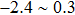dex. Following the definition of the two GC populations that 1P stars coincide with field stars having similar metallicity in Na abundance while 2P stars show Na overabundance, we adopted this set of relations as the criterion to separate 1P and 2P stars.

Making use of this field star criterion, we estimated the population fractions for 17 GCs whose stellar samples were analyzed homogeneously by Carretta et al. (, , ). An average 1P fraction of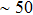% was found. Comparing to the 1P fractions derived by Carretta et al. () (chemically: [Na/Fe]min+0.3 dex) and by Milone et al. () (photometrically: chromosome map), our derived results are on average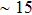% higher. However, the values by Carretta et al. () and Milone et al. () are consistent. We attribute the (dis)agreement between the studies to whether the diversity of GCs is taken into consideration, although the complexity of multiple stellar populations presented by a GC could lead to extra difficulty in deriving consistent population fractions among different population classification methods, as indicated by the cases of NGC 6388 and NGC 2808. Carretta et al. () and Milone et al. () both identified GC populations based on the stellar sample of individual GCs, so the specific 1P−2P separation criterion varied among GCs which could be a consequence of the GC diversity. However, the population separation criterion defined by field stars only depends on the GC metallicity, neglecting the various Na abundance distributions of GCs, which result from their different formation and evolution histories. Considering the case of NGC 6397 for which the two sets of methods show the most deviation in population fractions, we conclude that the population classification methods considering GC samples individually are more reasonable where the diversity of GCs can be taken into account. More caution should be exercised when comparing GC stellar samples to field stars when intending to identify 1P−2P GC stars.

However, in order to find a more efficient and accurate method to identify GC populations, further studies on the connection between chemical and photometric features of GC multiple populations are still in strong demand, as well as the relation between field stars and GCs.

 Carretta E. 2013 A&A 557 A128 Carretta E. Bragaglia A. Gratton R. G. et al. 2006 A&A 450 523 Carretta E. Bragaglia A. Gratton R. G. Lucatello S. Momany Y. 2007 A&A 464 927 Carretta E. Bragaglia A. Gratton R. G. et al. 2010 A&A 516 A55 Carretta E. Bragaglia A. Gratton R. G. et al. 2009 A&A 505 117 Decressin T. Baumgardt H. Charbonnel C. Kroupa P. 2010 A&A 516 A73 Decressin T. Meynet G. Charbonnel C. Prantzos N. Ekström S. 2007 A&A 464 1029 D’Ercole A. D’Antona F. Ventura P. Vesperini E. McMillan S. L. W. 2010 MNRAS 407 854 García-Hernández D. A. Mészáros S. Monelli M. et al. 2015 ApJ 815 L4 Gratton R. G. Carretta E. Claudi R. Lucatello S. Barbieri M. 2003 A&A 404 187 Krause M. Charbonnel C. Decressin T. Meynet G. Prantzos N. 2013 A&A 552 A121 MacLean B. T. Campbell S. W. De Silva G. M. et al. 2016 MNRAS 460 L69 Milone A. P. Marino A. F. Piotto G. et al. 2015 ApJ 808 51 Milone A. P. Piotto G. Renzini A. et al. 2017 MNRAS 464 3636 Renzini A. D’Antona F. Cassisi S. et al. 2015 MNRAS 454 4197 Schaerer D. Charbonnel C. 2011 MNRAS 413 2297 Ventura P. Di Criscienzo M. Carini R. D’Antona F. 2013 MNRAS 431 3642
Cite this article: Wang Yue, Zhao Gang, Li Hai-Ning. Classification of stellar populations in globular clusters. Res. Astron. Astrophys. 2017; 5:048.

### Refbacks

• There are currently no refbacks.